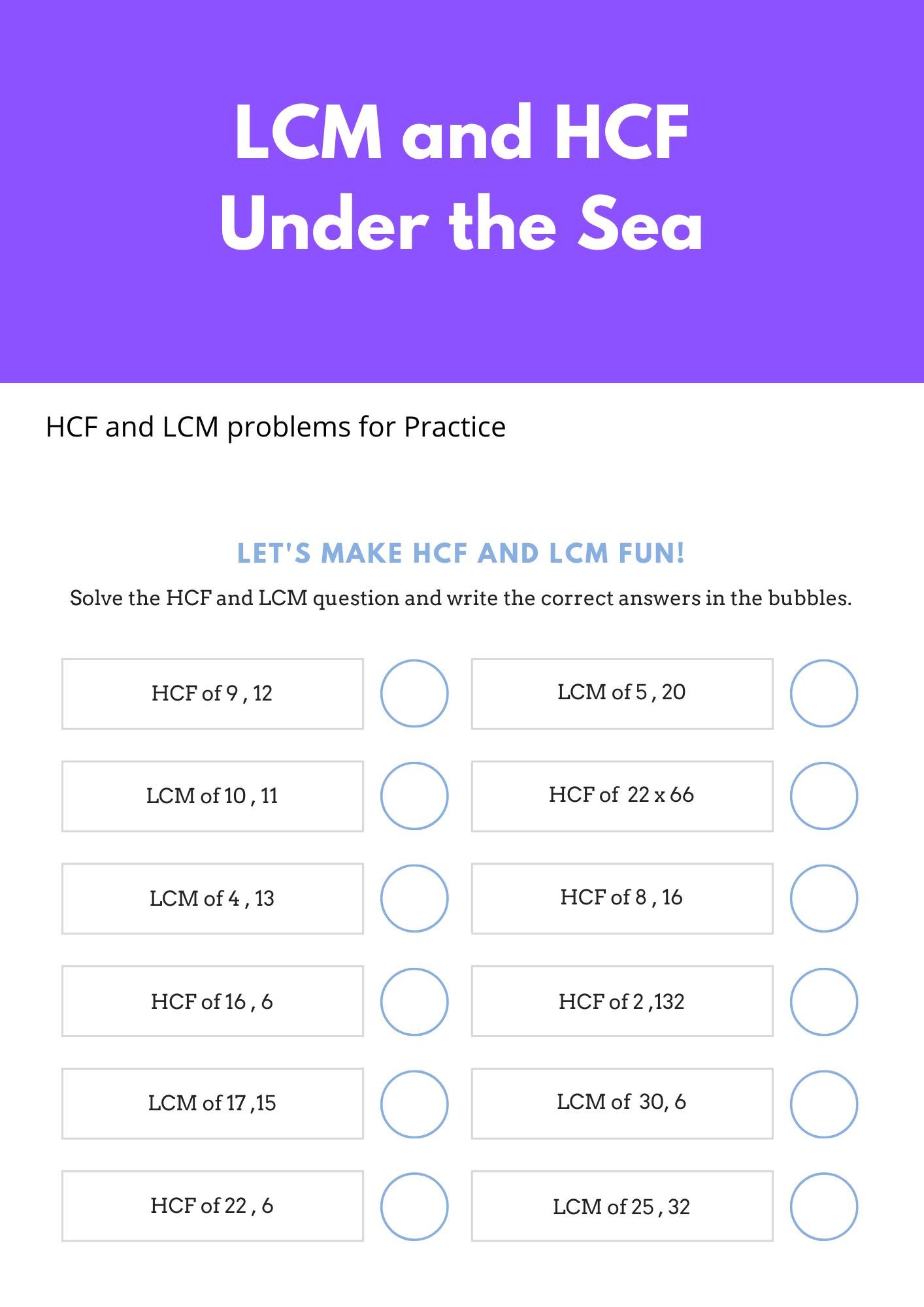# LCM and HCF questions for Class 6 Maths Playing With Numbers

In this page we have HCF and LCM questions for Class 6 Maths Playing With Numbers . Hope you like them and do not forget to like , social share and comment at the end of the page.
Question 1
Find the LCM of 12, 16, 24 and 36?

Question 2
Find the HCF of 70, 105, 175

Question 3
What are twin primes? Give four examples

Question 4
What is a prime triplet? Give an example.

Question 5
Arrange the prime factors of 1350 in descending order.

Question 6
Find the LCM of the following numbers:
a. 11 and 4
b. 125 and 5
c. 12 and 16
d. 8, 64

Question 7
Express 53 as the sum of three odd primes.

Question 8
In a morning walk, three persons step off together. Their steps measure 80 cm, 85 cm and 90 cm respectively. What is the minimum distance each should walk so that all can cover the same distance in complete steps?

Question 9
Find the least number which when divided by 12, 16 ,24 and 36 leaves a remainder 7 in each case.

Question 10
Find the multiples of 11 which is greater than 56 but less than 79

Question 11
Find the HCF of the following numbers:
(a) 11, 44
(b) 35, 42
(c) 128, 60
(d) 81, 63
(e) 36, 180
(f) 1152, 1664
(g) 70, 115, 175
(h) 91, 112, 49
(i) 18, 54, 81
(j) 12, 45, 85

Question 12
Find the LCM of the following numbers:
(a) 4,7,12,84
(b) 25,15,36
(c) 24,36,40
(d) 27,36
(e) 4,8,18
(f) 2,3,4,5,6,7

Practice HCF and LCM problems### Practice Question

Question 1 What is $\frac {1}{2} + \frac {3}{4}$ ?
A)$\frac {5}{4}$
B)$\frac {1}{4}$
C)$1$
D)$\frac {4}{5}$
Question 2 Pinhole camera produces an ?
A)An erect and small image
B)an Inverted and small image
C)An inverted and enlarged image
D)None of the above